# Introduction

The marcher package provides functions and tools for mechanistic range shift analysis decribed in Gurarie et al. (in press). The methods are designed to estimate parameters of range shifting, including coordinates of the centroids of (2 or 3) ranges, the times of initiation and duration of each shift, ranging areas and time scales of location and velocity autocorrelation. Because the estimates are likelihood based, there are several handy inferential tools including confidence intervals around all estimates and a sequence of hypothesis tests, including: (a.) What is the appropriate (highest) level of autocorrelation in the data? (b.) Is an estimated range shift significant? (c.) Is there a stop-over during the migration? (d.) Is a return migration a strict return migration?

In this vignette, I briefly introduce the family of range shift models and illustrate methods to simulate, visualize, estimate and conduct the hypothesis tests.

# Model description

The movement of an animal $${\bf z}(t)$$ is modeled as a possibly auto-correlated ranging process $${\bf r}(t)$$ around a mean process $${\bf m}(t)$$: ${\bf z}(t) = {\bf m}(t) + {\bf r}(t)$ where bold facing indicates 2D vectors representing an animal location on a plane in standard units, such as UTM easting and northing.

## Mean process

The mean component describes the range shift, thus its key parameters are the central location of each focal range and the timing (beginning and duration) of each transition. The simplest (and, practically, most useful) range shifting event is one with two ranges and no stop-overs, i.e. $${\bf m}(t)$$ shifts from one location to another over some transition duration $$\Delta t$$ beginning at some time $$t_1$$ ${\bf m}(t) = \begin{cases} {\bf m}_1 &\text{where} \,\, t < t_1 \\ {\bf m}_1 + ({\bf m}_2 - {\bf m}_1) {(t-t_1) \over \Delta t} &\text{where} \,\, t_1 < t < t_1 + \Delta t \\ {\bf m}_2 &\text{where} \,\, t > t_1 + \Delta t \end{cases}$ The mean process is defined by the six parameters $$\{x_1, y_1, x_2, y_2, t_1, \Delta t\}$$, where $$x_k$$ and $$y_k$$ are the coordinates of respective centroids $${\bf m}_k$$. The marcher package can estimate up to three range shifts.

## Ranging process

The ranging term $${\bf r}(t)$$ captures the spatial extent of the respective home ranges and features of the autocorrelation in the data. We consider several models for the ranging component, all of which are defined in continuous time, are spatially stationary, and can be meaningfully characterized by a typical area of use. The three possible ranging models are an uncorrelated two-dimensional white noise (WN), an Ornstein-Uhlenbeck position process (OU), which contains a first-order autocorrelation on the positions (see Dunn and Gipson 1977 and Blackwell 1997 for applications to animal home ranges), and a hierarchical Ornstein-Uhlenbeck velocity and position process (OUF) which additionally models autocorrelation in the velocities (Fleming et al. 2014). All of these processes can be characterized with respect to a circular area within which observations are expected to occur with 95% probability, which is the ranging area $$A$$.

## Summaries

The range shift models, combining the mean and ranging components, are denoted:

• Migratory white noise: $$\text{MWN}({\bf \mu}, A)$$ where $$\bf \mu$$ represents the mean process parameters described above;
• Migratory Ornstein-Uhlenbeck: $$\text{MOU}({\bf \mu}, A,\tau_z)$$ where $$\tau_z$$ is the autocorrelation time scale of crossing the home range;
• Migratory Ornstein-Uhlenbeck-Fleming: $$\text{MOUF}({\bf \mu}, A,\tau_z,\tau_v)$$ where $$\tau_v$$ is the autocorrelation time scale of velocity.

It is important to account for autocorrelations in modeling movements, and therefore a portion of any analysis is determining the appropriate level of autocorrelation, a step that is usually built-in in marcher packages. In practice, range shifting phenomemon nearly always occur at a much longer time scale than any possible velocity autocorrelation, and the MOUF models is highly unlikely to be selected. Selection of models is likelihood ratio or information criterion based. It is therefore important to keep track of the total number of parameters, which varies depending on the number of ranges and level of autocorrelation. For example, a two-range MWN has 7 parameters to fit ($$x_1, x_2, y_1, y_2, t_1, \Delta t, A$$), compared to a three-stage MOUF with 13parameters. In a basic range shift test, fitted models are compared to straightforward $$\text{WN}(A)$$, $$\text{OU}(A,\tau_z)$$, $$\text{OUF}(A,\tau_z,\tau_v)$$ models with 1, 2, and 3 parameters, respectively.

# Simulating range shift processes

Loading the package:

library(marcher)

R will prompt the user to install necessary dependencies as needed.

Simulating a range shift is not usually a primary objective of analysis, but understanding the functions that make it possible helps make the estimation process and output more clear. Also, it provides us with several datasets to illustrate and experiment with.

The simulate_shift function requires a vector of times of observation, a two-column vector of means of the process, and a list of ranging parameters. The mean of the process is first obtained by using the getMu function. First, specify the times of observations:

time <- 1:100

Next, the mean process parameters - which must be a named vector (i.e., the order does not matter, but the names x1, x2, y1, y2, t1, dt are necessary.):

mean.pars <- c(x1 = 0, y1 = 0, x2 = 10, y2 = 10, t1 = 45, dt = 10)

The getMu() function simulates the mean process, generating a two-column matrix of x and y coordinates:

Mean <- getMu(T = time, p.m = mean.pars)

The scan_track function is a convenient function for viewing a track, scattering the process as x-y, time-x and time-y lines. Note the rapid 20 time unit migration:

scan_track(time = time, x = Mean)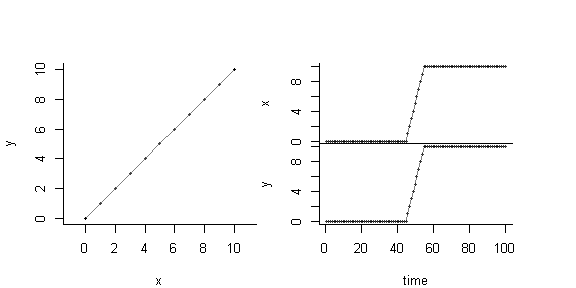To simulate the complete range shift message, we need only specify the ranging parameters, which are the ranging area A and, if needed, values of the autocorrelation time scales (tau_z and tau_v).

taus <- c(tau.z = 1, tau.v = 0.5)
area <- 50
SimTrack <- simulate_shift(T = time, tau = taus, mu = Mean, A = area)

The simulation data frame containes three columns:

head(SimTrack)
##   T          X          Y
## 1 1 -3.6459994 2.18233060
## 2 2 -2.6137021 1.29506637
## 3 3 -3.0184236 0.07590927
## 4 4 -1.0393050 1.46868807
## 5 5 -0.4471949 1.19663117
## 6 6 -1.8777918 0.98036577

Which are easily plotted with scan_track:

with(SimTrack, scan_track(time= T, x = X, y = Y))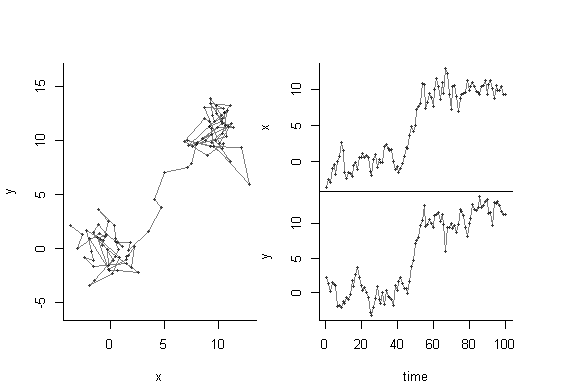Note, the scan_track() function will also automatically make this plot to a three-column data frame with names “T”,“X” and “Y”, so the above figure can be generated via: scan_track(SimTrack) as well.

It is now quick and easy to compare models with more or less position and velocity autocorrelation (especially with the magic of magrittr piping!):

MWN.sim <- simulate_shift(T = time, tau = NULL, mu = Mean, A = area) %T>% scan_track()
title("No Autocorrelation: MWN", outer = TRUE)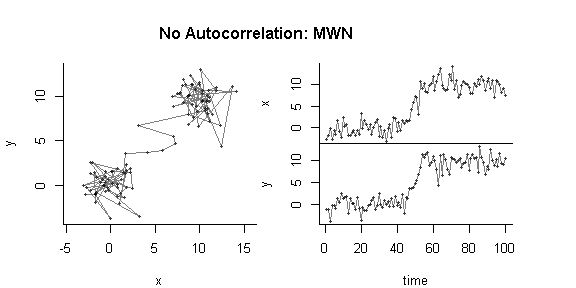MOU.sim <- simulate_shift(T = time, tau = c(tau.z = 5), mu = Mean, A = area)  %T>% scan_track()
title("Position Autocorrelation: MOU", outer = TRUE)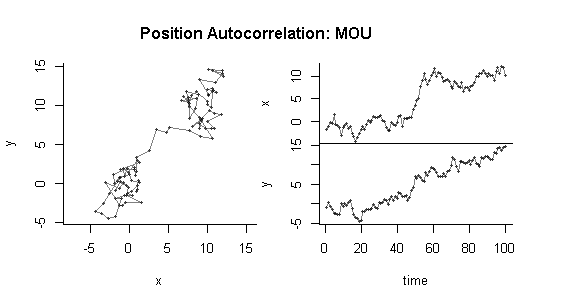MOUF.sim <- simulate_shift(T = time, tau = c(tau.z = 5, tau.v = 1), mu = Mean, A = area) %T>% scan_track
title("Position and Velocity Autocorrelation: MOUF", outer = TRUE)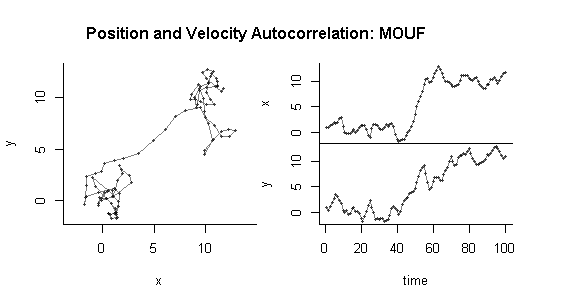Note that these processes can be simulated at random or arbitrary times of observation, e.g:

time.random <- sort(runif(100,0,100))
mean.random <- getMu(T = time.random, p.m = mean.pars)
MOUF.sim.random <- simulate_shift(T = time.random, tau = c(tau.z = 5, tau.v = 2), mu = mean.random, A = area) %T>% scan_track;
title("MOUF: random times", outer = TRUE)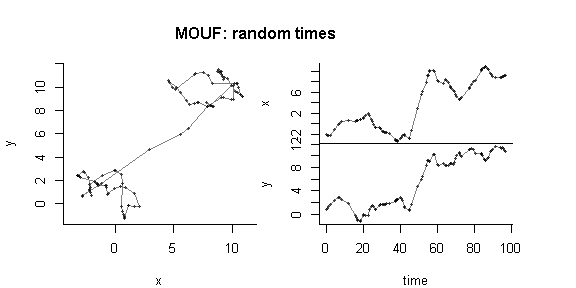This last version of data is perhaps the most difficult to estimate since it has two hierarchies of auto-correlation and irregular sampling.

Finally, a simulation with three ranges (two shifts), using the getMu_multi function to generate the mean process:

  p.m <- c(x1 = 0, x2 = 5, x3 = 10, y1 = 0, y2 = 5, y3 = 0, t1 = 40, t2 = 130, dt1 = 6, dt2 = 4)
A <- 20; T <- 1:200
Mu <- getMu_multi(T, p.m)
MOU.3range <- simulate_shift(T, tau=c(tau.z = 5), mu=Mu, A=A) %T>% scan_track
title("MOU: Three Ranges", outer = TRUE)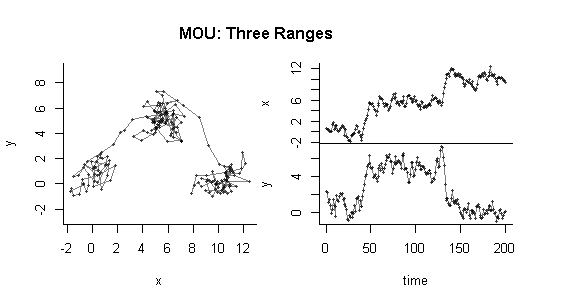We now have five simulated data sets to estimate range shifts with. Equivalent simulated tracks are stored in the marcher package, loaded via the command data(SimulatedTracks).

# Estimating range shifts

## … in one line of code

The key function for estimating range shifts is estimate_shift. There are many options in this function, and different ways to optimize for a specific task (or help it from breaking down), with further explanations in sections below. In the simplest implementation you simply run the function on time and location data:

MWN.fit <- with(MWN.sim, estimate_shift(T=T, X=X, Y=Y))
summary(MWN.fit)
## Range shift estimation:
##
## 100 observations over time range 1 to 100.
## 2 ranges (one shift) fitted using the AR method.
##
## Initial shift parameters obtained from quickfit:
##      t1      dt      x1      y1      x2      y2
## 46.5200  4.9500  0.1137  0.4645  9.8200  9.4230
## The AIC-selected ranging model is WN.
##
## Parameter Estimates:
##          p.hat     CI.low     CI.high
## A  45.86415869 36.8747836 54.85353380
## t1 43.99926918 43.9099271 44.08861125
## dt 10.44845740  9.8963728 11.00054198
## x1 -0.25554574 -0.4577088 -0.05338273
## y1  0.04525516 -0.1569079  0.24741818
## x2  9.93171618  9.7347831 10.12864922
## y2  9.60101744  9.4040844  9.79795048
##
## log-likelihood: -371.8; AIC: 757.7; degrees of freedom: 7

The output can be visualized readily with the plot.shiftfit method:

plot(MWN.fit)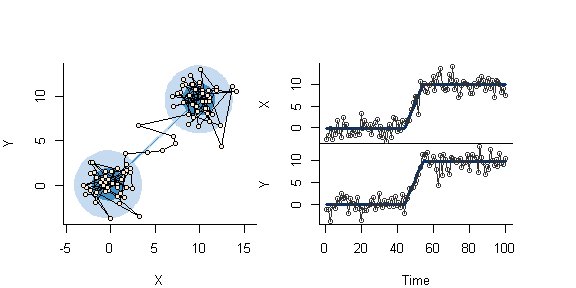Note that in this visualization, the area circles are (dark to light) the 50% and 95% areas of use, whereas the dark and light blue lines in the time series figure reflect the confidence intervals around the estimated means, which are rather narrow.

Here is the same procedure applied to the three step process:

with(MOU.3range, estimate_shift(T=T, X=X, Y=Y, n.clust = 3)) %>% plot
## Calculating area directly (time range much greater than time scale).
## Using bootstrap for confidence intervals around the variance parameters.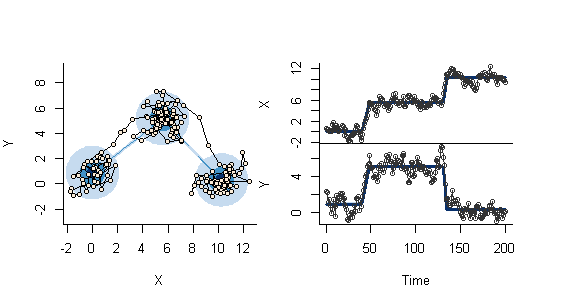This is the most straightforward black-box application of the range shift estimation. Below, we go into more detail as to the meaning of the output and the various way to specify the methods and models.

## Details of the output

The fitted object is of a (marcher specific) shiftfit class object, the summary output of which provides a lot of useful information. Most obviously, all of the parameter estimates and confidence intervals (which should be pretty close to the true values) and the log-likelihood and AIC of the model. A few important additional bits:

1. Because we did not provide a ranging model, the algorithm did an AIC based selection and decided that the WN model is most suitable for the ranging (residuals). The model can be specified with the model argument (one of “WN”, “OU” or “OUF”, case insensitive).

2. Because we did not provide initial parameter values (argument p.m0 - a named list of parameter values), the algorithm used the quickfit() function to find centroids and reports those in the summary output (see below). This generally works well enough for clear range shifts. There are functions available to (interactively) simplify the process of selecting initial parameter value guesses.

3. Because we did not provide a method, the fit was performed using the AR equivalence for estimation (see paper for explanation). This method is fast, but generally unsuitable for irregularly sampled data (unless the ranging model is white noise) and for OUF ranging models. IN these cases, the maximum likelihood method is preferable. The method can be specified with the method argument (one of ar or like - also case insensitive).

## Seeding parameters

Without initial mean parameter given, estimate_shift uses a simple k-means clustering to find two (or three) clusters in data to initialize the fitting with the quickfit function. This function does a reasonable job at estimating the mid-point of the migrations from the clustering, but has no way of estimating the duration, which needs to be entered manually.

(p.m0 <- with(MWN.sim, quickfit(T,X,Y, dt = 1)))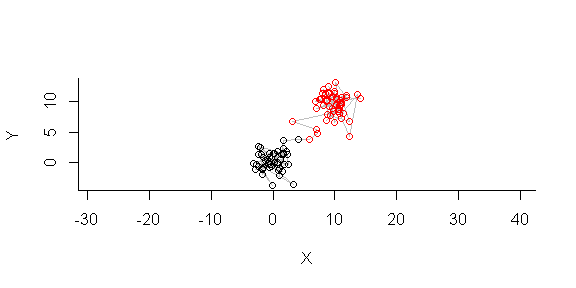##          t1          dt          x1          y1          x2          y2
## 47.50000000  1.00000000 -0.06974061  0.26418434  9.61628490  9.26361918

The quickfit function will also generally work for three ranges:

(p.m0 <- with(MOU.3range, quickfit(T,X,Y, dt = 1, n.clust = 3)))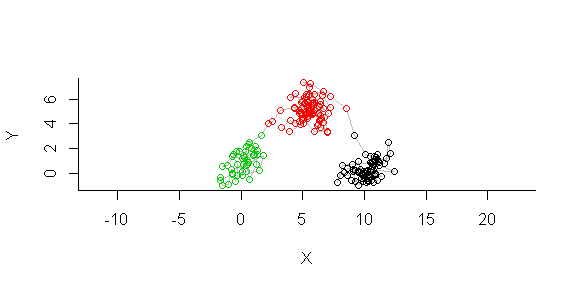##          x1          x2          x3          y1          y2          y3
##   0.1335115   5.5143083  10.2271261   0.9142110   5.0893057   0.3208889
##          t1          t2         dt1         dt2
##  43.0000000 132.0000000   1.0000000   1.0000000

Because of the slightly cryptic operation of the kmeans function underlying quickfit, the three cluster is not quite as reliable as the two shift version, providing a slightly different result each time it is run. Some supervision is always preferable.

A second option for obtaining initial parameter values is to use the locate_shift function, which uses R’s interactive locator function and prompts the user to click on the track scan to obtain initial values. Typically this works rather well:

(p.m0 <- with(MWN.fit, locate_shift(T,X,Y, dt = 1)))

In a typical workflow, if you’d like to seed your estimation with more reliable guesses than the quickfit values, you would just pass the output of locate_shift to the p.m0 paramter of estimate_shift:

estimate_shift(T, X, Y, p.m0 = p.m0)

## Residual analysis and autocorrelation model selection

One of the outputs of the fitted model object is a vector of residuals. These residuals capture all of the ranging behavior (and deviations from the migration track during migration). It is in the analysis of these residuals that a ranging area and a selection between autocorrelation models (WN / OU / OUF) occurs.

Below, we visualize the three residual vectors from fits of the the three single-shift models. Note that we prefer using the likelihood method for the MOU and MOUF simulations and - in order to speed up the fitting - we pre-assigned the fits to the white noise (MWN) model, since we are interested in analyzing the residuals.

## Warning in par(mfrow = c(1, 3), bty = "l", echo = -1): "echo" is not a
## graphical parameter
data(SimulatedTracks)
MWN.res <- with(MWN.sim, estimate_shift(T, X, Y, model = "wn"))$Z.res MOU.res <- with(MOU.sim, estimate_shift(T, X, Y, model = "wn"))$Z.res
MOUF.res <- with(MOUF.sim, estimate_shift(T, X, Y, model = "wn"))$Z.res plot(MWN.res, asp=1, type="o") plot(MOU.res, asp=1, type="o") plot(MOUF.res, asp=1, type="o")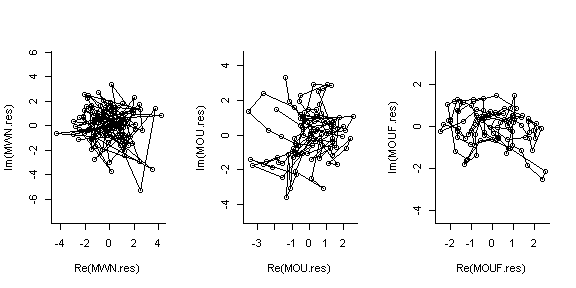These residuals all have similar spatial scales and circular symmetry, but the increasing autocorrelation in these residuals is visually quite clear. The selectModel function determines which model is most appropriate for each of these residuals: selectModel(MWN.res, T = MWN.sim$T, method = "like", showtable = TRUE)
## $model ##  "wn" ## ##$aic
##      wn      ou     ouf
## 747.689 749.689 751.689
selectModel(MOU.res, T = MOU.sim$T, method = "like", showtable = TRUE) ##$model
##  "ou"
##
## $aic ## wn ou ouf ## 696.8643 543.7860 545.7862 selectModel(MOUF.res, T = MOUF.sim$T, method = "like", showtable = TRUE)
## $model ##  "ouf" ## ##$aic
##       wn       ou      ouf
## 595.2874 329.1360 291.6746

These determinations (all of which happen to be correct) are made based on the reported AIC values. This function is run within the estimate_shift function by default, but most efficiently if you are analyzing multiple individuals sampled at similar rates, one determination (most commonly - OU or WN) is enough to apply to all individuals.

Once a model is selected, the getTau function estimates the values of the time scale parameters (if needed)

getTau(MOU.res, T = MOU.sim$T, model = "ou", CI = TRUE)[c("tau.hat","tau.CI")] ##$tau.hat
##   tau.z
## 3.44638
##
## $tau.CI ## tau.z ## low 2.212200 ## high 5.369106 getTau(MOUF.res, T = MOUF.sim$T, model = "ouf", CI = TRUE)[c("tau.hat","tau.CI")]
## $tau.hat ## tau.z tau.v ## 2.245709 1.010063 ## ##$tau.CI
##          tau.z    tau.v
## low  0.8089877 0.000000
## high 6.2339723 2.245709

For the OUF, which is the most layered autocorrelated model, the estimates of the time scales can be rather imprecise, with wide confidence intervals, especially with relatively limited data. Estimating the velocity autocorrelation is a difficult task, and for most migration and range-shifting process, not particularly relevant because the process occurs at a time scale so much greater than any velocity autocorrelation scale.

A reminder: these functions all run under the hood of estimate_shift, but can be useful for exploring aspects of the data.

# Example with roe deer data

## Entire track

There is one deer, Michela, in the package:

data(Michela)
with(Michela, scan_track(time = time, x = x, y = y))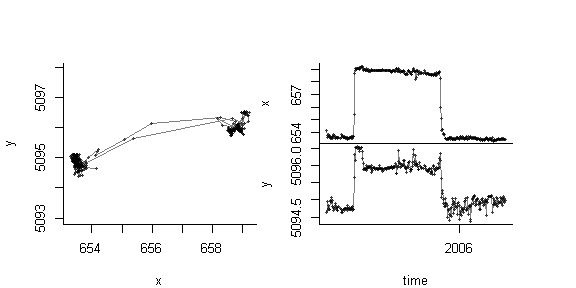Over the period of study, between February 2005 and April 2006, there is a clear seasonal migration to a summering ground and a return.

We can fit a three range model to the data. Note: for the time being, the estimation routine can only handle numeric time objects. Time data that are stored as POSIX (the standard time object) need to be converted to a unit like days or hours from an initial time. For example, in the case of Michela, the day column was generated as:

# first day of first year
day1 <- lubridate::ymd(paste(min(lubridate::year(Michela$time)), 1, 1)) # find time difference and round (and make numeric) days <- as.numeric(round(difftime(Michela$time, day1, unit = "day")))

The initial guesses are best obtained using the interactive locate_shift function, which was used to obtain the values entered below.

# p.m0 <- with(Michela, locate_shift(time = day, x = x, y = y, n.clust = 3))
p.m0 <- c(x1 = 653.6,  x2 = 658.85, x3 = 653.8,
y1 = 5094.8, y2 = 5096,   y3 = 5094.8,
t1 = 118, t2 = 318, dt1 = 10.5, dt2 = 15.8)
Michela.fit <- with(Michela,
estimate_shift(day, x, y, n.clust = 3, model = "ou", method = "like", p.m0 = p.m0))
## Calculating area directly (time range much greater than time scale).
plot(Michela.fit)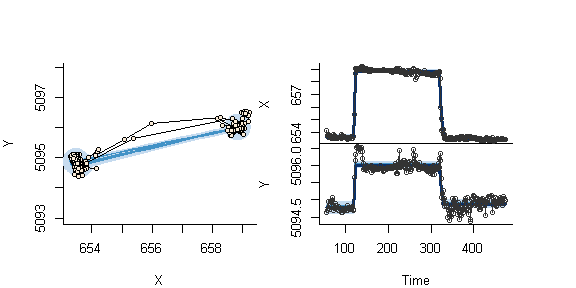The time variable here is day of year from January 1 of the first year of observation. We can convert those results to dates using functions in lubridate:

lubridate::ymd("2005 1 1") + lubridate::ddays(Michela.fit$p.hat[c('t1','t2')]) ##  "2005-05-01 23:48:16 UTC" "2005-11-15 09:11:26 UTC" So the first migrations occurred on April 29 and lasted 6.9 days (95% CI 5.5-8.15), while the return migration occurred on November 17 and lasted about 5 days (3.9 - 6). ## First migration It appears from the figure above that Michela settled into the summer territory after first exploring a region a bit to the north. We can fit the early stages of the migration as well as below: # p.m0 <- with(subset(Michela, day < 200), locate_shift(time = day, x = x, y = y, n.clust = 3)) p.m0 <- c(x1 = 653.6, x2 = 659.13, x3 = 658.96, y1 = 5094.8, y2 = 5096.5, y3 = 5095.9, t1 = 120.5, t2 = 138.7, dt1 = 4, dt2 = 4.7) Michela.fit.early <- with(subset(Michela, day < 200), estimate_shift(day, x, y, n.clust = 3, model = "ou", method = "like", p.m0 = p.m0)) ## Calculating area directly (time range much greater than time scale). plot(Michela.fit.early)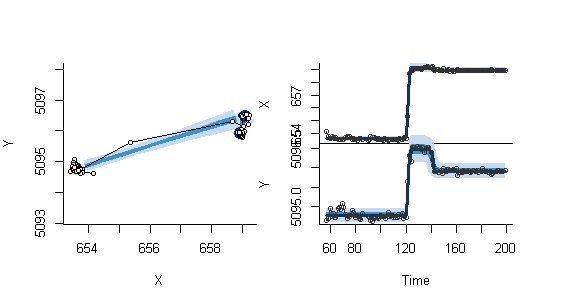summary(Michela.fit.early) ## Range shift estimation: ## ## 113 observations over time range 57 to 199. ## 3 ranges (2 shifts) fitted using the LIKE method. ## The fitted ranging model is OU. ## ## Parameter Estimates: ## p.hat CI.low CI.high ## A 0.1338783 0.1091937 0.158563 ## tau.z 1.1644557 0.8046559 1.685139 ## x1 653.6120513 653.4168762 653.807226 ## x2 659.0808641 658.7259787 659.435750 ## x3 658.9176003 658.7124775 659.122723 ## y1 5094.7724276 5094.5772525 5094.967603 ## y2 5096.4730558 5096.1181703 5096.827941 ## y3 5095.9071557 5095.7020330 5096.112279 ## t1 119.9390514 119.4775433 120.400559 ## t2 139.7497665 135.4537010 144.045832 ## dt1 3.3032095 2.4882910 4.118128 ## dt2 3.1622144 0.0000000 12.258716 ## ## log-likelihood: 255.4; AIC: -486.8; degrees of freedom: 12 Be will use both of these results to perform some hypothesis test in the next section. Note, incidentally, that the time-scale of position auto-correlation of Michela’s movements is about 1.2 days, indicating that her ranging movements occur, generally, over a time span greater than one day. # Further statistics and hypothesis tests The likelihood formulation allows for several hypothesis tests to be performed. These can be useful in cases where there are many individuals with some ambiguous cases and some criterion is necessary for classifying migration behavior. ## Migration distance and range shift index Useful measures for quantifying a range shift are the distance between the centroids of the respective ranges, and the range shift index (RSI) as described in Gurarie et al. (in press). This RSI is simply the ratio between the distance between ranges and the diameter of the 95% ranging area. The function getRSI obtains this index (with confidence intervals) from a given fit. Below, these statistics for Michela’s first migration: getRSI(Michela.fit, 1, 2) ## p.hat CI.low CI.high ## D 5.369496 5.164471 5.587879 ## RSI 6.143766 5.766805 6.590245 A distance of only 5.4 km, and a range shift index of 6.1. Compare those with the return (which is nearly identical) and the difference between the first and final ranges: data.frame(FirstToSecond = getRSI(Michela.fit, 1,2)[,1], SecondToThird= getRSI(Michela.fit, 2,3)[,1], FirstToThird = getRSI(Michela.fit, 1,3)[,1]) ## FirstToSecond SecondToThird FirstToThird ## D 5.369496 5.473727 0.1645583 ## RSI 6.143766 6.263027 0.1882872 The results are unsurprising, but nice to quantify. ## Range shift test The RSI is, essentially, an effect’’ size of a migration process. A fundamental test of significance for a range shift is provided by comparing a model with and without migration. As a relatively challenging example, we can take the randomly sampled MOUF simulation: FIT <- with(MOUF.sim.random, estimate_shift(T, X, Y, method = "like", model = "ouf")) ## Calculating area from time scale constants due to insufficient time range. plot(FIT)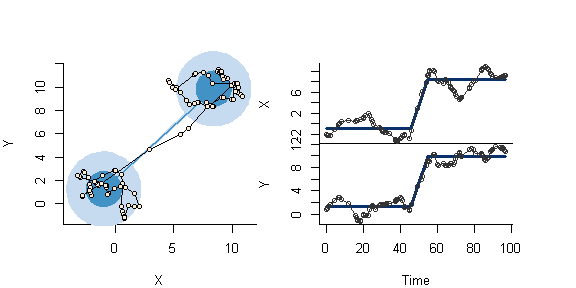The test_rangeshift function takes a fitted range shift object and performs the test, outputting an AIC table as well: test_rangeshift(FIT) ## ll k aic ## with shift 15.83279 5 -21.66558 ## without shift 40.96015 9 -63.92029 ## ## l.r.t.: -50.3 with 4 degrees of freedom, p-value: 1 ## Tough call - there's disagreement in the criteria. Clearly, there is a significant shift, with a very small p-value. We contrast this with a range shift fit of the first 45 locations, where (as simulated) there shouldn’t be a range shift at all. FIT2 <- with(MOUF.sim.random[1:45,], estimate_shift(T, X, Y, method = "like", model = "ouf")) ## Calculating area from time scale constants due to insufficient time range. plot(FIT2) ## Some confidence intervals around the timing parameters are infinitely wide. I'll plot something fat but not quite THAT wide. The range shift model might not be appropriate.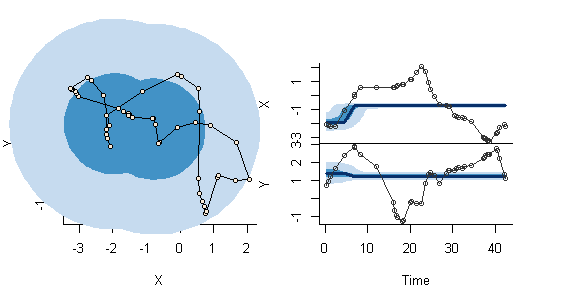test_rangeshift(FIT2) ## ll k aic ## with shift 27.20963 5 -44.41926 ## without shift 24.98357 9 -31.96715 ## ## l.r.t.: 4.45 with 4 degrees of freedom, p-value: 0.108 ## There probably wasn't a range shift. The output is unambiguous about there being no significant range shift in these data. ## Return test Michela performed what is most likely a return migration at the end of 2005 to what was her winter ranging grounds from the previous year. This hypothesis can be tested by comparing the more complex fitted model of three ranges against a null model where the third range is the original range. The test_return function does just that. test_return(Michela.fit) ## ll df aic ## two range with return 390.8059 10 -801.6118 ## three ranges 392.0192 12 -760.0385 ## ## l.r.t.: 2.43 with 4 degrees of freedom, p-value: 0.2972 ## The second migration was most likely a return migration. Note that the default for this test is the likelihood method, which is somewhat slower. Very similar results are obtained using the AR equivalence, e.g.: test_return(Michela.fit, method = 'ar') ## Stopover test In some cases, it might be interesting to know whether or not a stopover occurred, or if two of three potential ranges could “statistically” be pooled into one. For example, in the second Michela example above, the second and third ranges were rather close to each other, and a two range model might be more parsimonious. Note that the likelihood and the AIC of the three range model are: c(logLik(Michela.fit.early), AIC(Michela.fit.early)) ## ll aic ## 255.3839 -486.7678 A fitted two range model for that subset yields: Michela.fit.early2 <- with(subset(Michela, day < 200), estimate_shift(day, x, y, n.clust = 2, model = "ou", method = "like")) ## Calculating area from time scale constants due to insufficient time range. c(logLik(Michela.fit.early2), AIC(Michela.fit.early2)) ## ll aic ## 216.4880 -416.9759 Clearly, the AIC of the three range model is much lower than the AIC of the two range model. This process can be wrapped up into a single line of code: test_stopover(Michela.fit.early) ## Calculating area from time scale constants due to insufficient time range. ## ll aic ## with stopover 216.4889 -416.9778 ## without stopover 255.3839 -486.7678 ## ## l.r.t.: 77.8 with 4 degrees of freedom, p-value: 5.551e-16 ## A three range model (with stopover) is a better model. # Additional feature: net square displacement A net-squared displacement analysis (NSD analysis) is an alterantive method to characterize dispersal or range-shifting behavior. We include a function (fitNSD) to fit a migration curve to a net-squared displacement, following the method outlined in Börger et al. (2012). The method also tests for the significance of the displacement. The example below is from the fitNSD help file. # set initial parameters A <- 20; T <- 1:100; tau <- c(tau.z = 2, tau.v = 0) # simulate, fit and test clear disperal Mu <- getMu(T, c(x1 = 0, y1 = 0, x2 = 4, y2 = 4, t1 = 40, dt = 20)) XY.sim <- simulate_shift(T, tau = tau, Mu, A=A) with(XY.sim, scan_track(time = T, x = X, y = Y))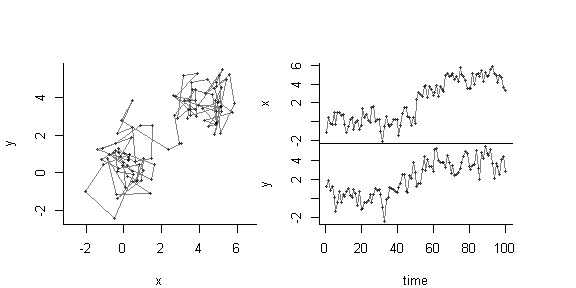with(XY.sim, fitNSD(T, X, Y, plotme=TRUE))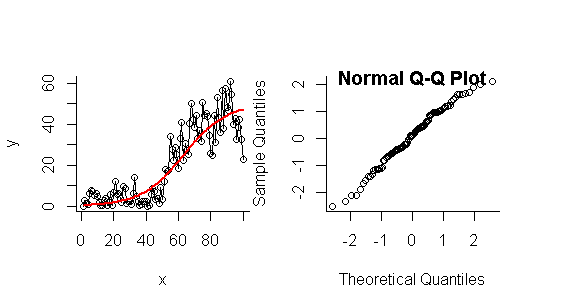##$estimate
##         a         b         c         s
## 60.832643 50.500000  1.000000  2.285145
##
## $test ## LL AIC LL.null AIC.null p.value ## -144.8249 297.6499 -224.0368 452.0736 0.0000 # simulate, fit and test no disperal Mu <- getMu(T, c(x1 = 0, y1 = 0, x2 = 0, y2 = 0, t1 = 40, dt = 20)) XY.sim <- simulate_shift(T, tau = tau, Mu, A=A) with(XY.sim, scan_track(time = T, x = X, y = Y))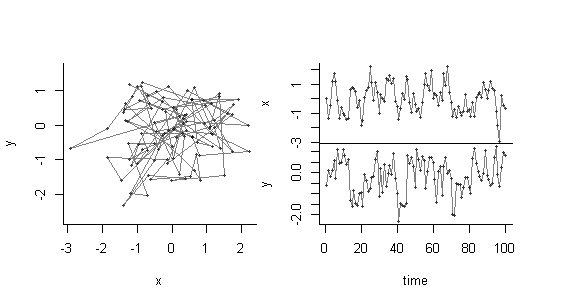with(XY.sim, fitNSD(T,X,Y, plotme=TRUE))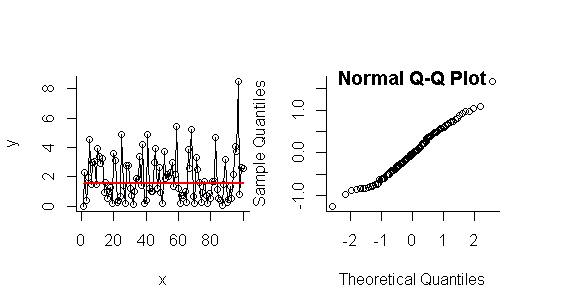##$estimate
##          a          b          c          s
##  8.4914201 50.5000000  1.0000000  0.5702801
##
## \$test
##          LL         AIC     LL.null    AIC.null     p.value
## -85.2285861 178.4571723 -85.2310950 174.4621900   0.9974943

The qq-plot diagnostics help confirm that the Gaussian model used to fit the square root of NSD sufficiently stabilized the variances around the ordinary least squares fit.